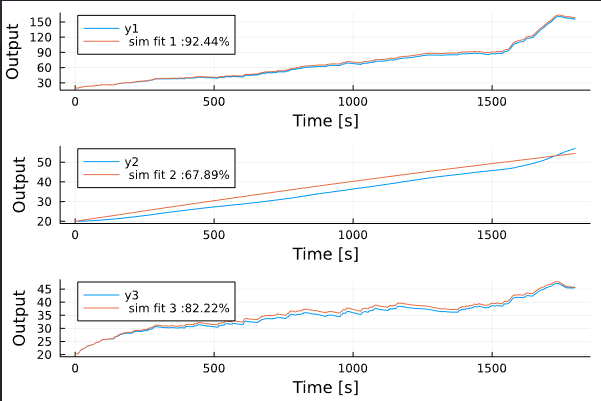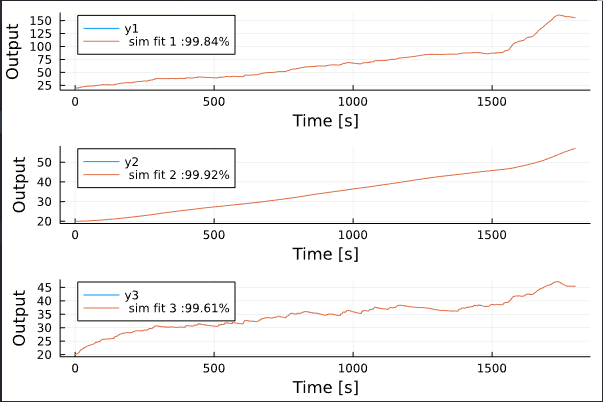# Parameter Estimation of a 3 node thermal network

Im trying to formulate a parameter estimation problem to estimate the Resistance and Capacitance values of a 3 node thermal network. The thermal network is in the form of DAE with a mass matrix and exogenous inputs. I already have the temperature values of my 3 nodes as an interpolation function and im using it to estimate the loss of the then use Optimization.solve to solve the problem.

using DifferentialEquations, Interpolations,
Optimization, OptimizationPolyalgorithms, SciMLSensitivity,
Zygote, OptimizationOptimisers, OptimizationNLopt
using Plots
import XLSX

function thermal(du, u, p, t, P1, P2)
T1, T2, T3 = u
R_12, R_32, R_35, R_24, T4, T5 = p
du = T2/R_12 - T1/R_12 + P1(t);
du = T2/R_12 + T4/R_24 + T3/R_32 - T2*(1/R_12+1/R_24+1/R_32);
du = T2/R_32 + T5/R_35 - T3*(1/R_32+1/R_35) + P2(t);
end

p=[0.5,0.2,0.1,0.05,30,30,1000,3000,2000];
M = [p[end-2] 0 0
0 p[end-1] 0
0 0 p[end]]

sh = xf[“Exp1”]
p1 = sh[“C5:C1804”]
p2 = sh[“D5:D1804”]
t1 = sh[“H5:H180004”]
t2 = sh[“I5:I180004”]
t3 = sh[“J5:J180004”]
d = 0.01:0.01:1800;
t = 1:1:1800;
tsteps = 0.1:0.1:1800
P1=LinearInterpolation(t,vec(p1’));
P2=LinearInterpolation(t,vec(p2’));
T1_s=LinearInterpolation(d,vec(t1’));
T2_s=LinearInterpolation(d,vec(t2’));
T3_s=LinearInterpolation(d,vec(t3’));

#experimental power and temperature values

u=[20,20,20];
tspan = (1,1800);
f = ODEFunction(thermal, mass_matrix = M)
prob_mm = ODEProblem(f, u, tspan, p)
sol = solve(prob_mm, Rodas5P(), saveat = 0.01, abstol=1e-8, reltol=1e-8)
plot(sol);

function loss(p)
sol = solve(prob_mm, Rodas5P(), p=p, saveat = 0.01, abstol=1e-8, reltol=1e-8)
T1_hat = [u for u in sol.u]
T2_hat = [u for u in sol.u]
T3_hat = [u for u in sol.u]

``````T1_model = T1_s(sol.t)
T2_model = T2_s(sol.t)
T3_model = T3_s(sol.t)

loss1 = sum(abs2, T1_model .- T1_hat)
loss2 = sum(abs2, T2_model .- T2_hat)
loss3 = sum(abs2, T3_model .- T3_hat)

loss = loss1+loss2+loss3
return loss, sol
``````

end

callback = function (p, l, pred)
display(l)

# display(plt)

``````# Tell Optimization.solve to not halt the optimization. If return true, then
# optimization stops.
return false
``````

end

optf = Optimization.OptimizationFunction((x, p) → loss(x), adtype)
optprob = Optimization.OptimizationProblem(optf, p)

result_ode = Optimization.solve(optprob, PolyOpt(), callback=callback, maxiters = 100)

I have tried with different AD solvers and different algorithms but this keeps throwing me
“ERROR: gradient of 180000-element extrapolate(scale(interpolate(::Vector{Any}, BSpline(Linear())), (0.01:0.01:1800.0,)), Throw()) with element type Float64:”
3node_thermal_network.jl (2.2 KB)

Hello and welcome to the communityCould you post the data file, such that people can run your code and reproduce the error you are seeing?

Hi Fredrik,
The data file i have in a .xlsx file and i am not able to upload it, is there any other way i could possibly share the file ?

Any standard file sharing service I guess, google drive, dropbox etc.?

Here’s an example that uses a simple implementation of the prediction-error method. I call the function `nonlinear_pem`, even though this system is actually linear in the state and inputs.

``````function thermal(u, input, p, t)
P1, P2 = input
T1, T2, T3 = u
R_12, R_32, R_35, R_24, T4, T5 = p
SA[(T2/R_12 - T1/R_12 + P1) / p[end-2]
(T2/R_12 + T4/R_24 + T3/R_32 - T2*(1/R_12+1/R_24+1/R_32)) / p[end-1]
(T2/R_32 + T5/R_35 - T3*(1/R_32+1/R_35) + P2) / p[end]]
end

sh = xf["Exp1"]
p1 = Float64.(sh["C5:C1804"][:])
p2 = Float64.(sh["D5:D1804"][:])
t1 = Float64.(sh["H5:H180004"][:])
t2 = Float64.(sh["I5:I180004"][:])
t3 = Float64.(sh["J5:J180004"][:])
d = 0.01:0.01:1800;
t = 1:1:1800;
plot(t, u, layout=(5,1))
plot!(d, y, sp=(3:5)')

Ts = 1
t = 1:Ts:1800

## experimental power and temperature values
u = [p1 p2] # Replace with input  measurement data here
y = [t1 t2 t3] # Replace with output measurement data here

d = iddata(y[1:100:end, :]', u', Ts) # Use every 100th sample of the outputs

# Initial guess
p0 = [0.5,0.2,0.1,0.05,30,30,1000,3000,2000]
u0 = SA[20.0,20,20]

R1 = 1e-4I(3) # Tuning parameters for the internal Kalman filter
R2 = 0.1I(3)
nx, nu, ny = 3, 2, 3 # Number of state variables, inputs and outputs

discrete_dynamics = SeeToDee.Rk4(thermal, Ts) # Discretize the continuous-time dynamics
measurement = (u, input, p, t) -> u # Measurement function is the identity

model = ControlSystemIdentification.nonlinear_pem(
d,
discrete_dynamics,
measurement,
p0,
u0,
R1,
R2,
nu;
optimize_x0 = false,
lower = 0.1*p0, # Constrain the search space to be within 10% of the initial guess
upper = 10*p0,
)

simplot(model, d, layout=(3, 1))
``````The estimated parameters are available as

``````julia> model.p
9-element Vector{Float64}:
0.5042616495643081
0.22669213158156562
0.10462125464969055
0.447811612771852
300.0
29.79321313071231
1006.2410623845433
30000.0
2004.2052737272784
``````

The model fit is not perfect, in particular, there seem to be some problem with modeling the second output. This can be either due to missing dynamics in the model, due to the gradient-based optimizer getting stuck in a local minimum, or due to me constraining the parameters to be within 0.1-10x of the initial guess. You probably have better insight into what values are reasonable hereIf the problem is due to a local minimum, you could try some form of global optimizer instead. However, this is not supported by the function `nonlinear_pem`. @SebastianM-C might have suggestions that can handle local minima better.

1 Like

BTW, a black-box linear model of order 4 fits the data perfectly:

``````bbmodel, x0 = newpem(d, 4, zeroD=true, focus=:simulation)
simplot(bbmodel, dd, x0, layout = (3, 1))
``````Is the data simulated?

1 Like

This is really great. Actually i have been using Matlab’s global optimization toolbox to estimate the values and been getting decent results but i haven’t tried implementing the same in Julia yet.